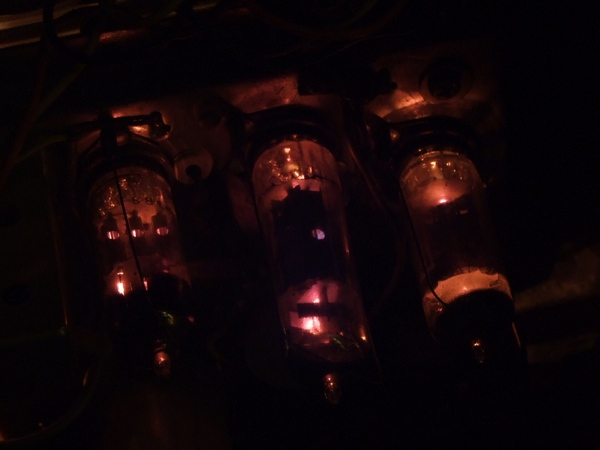Vervainglobal is all about challenging technology to make it do the things you want it to do. With heavy focus on low cost, minimal tools and simplicity, virtually anyone can learn how to create and modify tech to their needs. Articles here focus on both hardware and software hacking, and will teach you how it all works through practical learning. Please note: Third party cookies are used by vervainglobal. By continuing to browse this site, you agree to their use. Learn more here.

## Basic electrical principles explained

This page will teach you the fundamental basics of how electricity works. It will help you to understand the relationship and differences between Voltage, Current and Power.### Measuring electricity

You'll almost certainly have heard of Volts, Amps and Watts. These units of measurement are all ways of monitoring electricity in some way. Despite that, they all measure something different. Knowing the importance and difference of these units will give you a whole new understanding of electricity, and how some of your electrical appliances work.

### Units of measure

First up is the Volt. Everyone's heard of this one. The name was coined by the physicist Alessandro Volta in 1792, shortly before he invented the first battery. A "volt" is infact a measurement of potential difference. That is, it measures the difference in electrical charge between two conductors. If you were to visualise electricity as a liquid, potential difference is like pressure. The pressure of 2 volts would be twice that of the pressure of 1 volt. The symbol for voltage is the capital "V"

Next is Current. Current is measured in amperes, or amps. This one was named after the French mathematician, Andre-Marie Ampere. If voltage is like pressure, current is like flow. As electricity flows faster through a cable, current rises. The symbol for current is the capital letter "I".

Then we have Power. Power is a measurement of how much "work" a source of energy is performing. When a source of energy changes form, for example electrical energy being turned into heat and light energy by a light bulb, we can measure how fast that energy is changing form. Power can be measured in several different units, including the Joule and the Horsepower. In electricity, the Watt is by far the most commonly used. This one was named after the Scottish engineer, James Watt. The symbol "P" is often used to represent power, but you will sometimes see the letter "W".### Adding it all together

On their own, these units don't tell us a lot. More often than not, we find ourselves with an electrical appliance or device where we know it's voltage and wattage, but need to know the current. A good example is when you need to check what size fuse we need for, say, the washing machine. Thankfully, there is a simple equation we can use to find our answer. Enter the power triangle (see image right). With this simple equation, we can discover the missing unit from the two units we already know.

Let's say our appliance is a washing machine. On the little rating plate, it tells us that it needs 240 volts, and uses up to 2500 watts. We need to put a fuse in the plug, but are not sure which one is most appropriate. Looking at our triangle, we see Power is at the top, with voltage and current side by side underneath. So, with our washing machine, we would divide 2500 by 240. With equation triangles, the top number must be divided by the bottom number, and never the other way around. If the two numbers are side by side, you just need to multiply them together.

So 2500 / 240 = 10.41 amps. A 10 amp fuse would be just a little too small. So the fuse of choice would be the 13A one. With this simple equation triangle, any two numbers can be used to find out the third. For example, you might have a 3 amp fuse and want to know how much power can be safely drawn through it. So we would do: 240 volts x 3 amps = 720 watts. Being able to find the missing unit allows us to perform many tasks, like selecting the right fuse, or choosing the correct cable size for our project. Once you have a good understanding of the information in this article, you will begin to understand the unique behaviours and characteristics of electricity. The next article will explain to you the principal of resistance, and how it is so important to almost every electrical appliance ever built.

Part 2 - Resistance in electricity